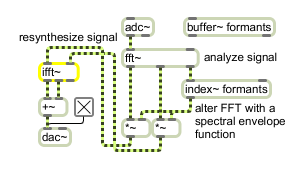# ifft~

Inverse fast Fourier transform

## Description

ifft~ performs an Inverse FFT (inverse fast Fourier transform) on an input signal.

## Arguments

Name Type Opt Description
IFFT-points (samples) int opt The first argument specifies the number of points (samples) in the IFFT. It must be a power of two. The default number of points is 512. The second argument specifies the number of samples between successive IFFTs. This must be at least the number of points, and must be also be a power of two. The default interval is 512. The third argument specifies the offset into the interval where the IFFT will start. This must either be 0 or a multiple of the signal vector size. ifft~ will correct bad arguments, but if you change the signal vector size after creating an ifft~ and the offset is no longer a multiple of the vector size, the ifft~ will not operate when signal processing is turned on.

## Messages

 signal In left inlet: The real part of a complex signal that will be inverse transformed. In right inlet: The imaginary part of a complex signal that will be inverse transformed. If signals are connected only to the left inlet and left outlet, a real IFFT (inverse Fast Fourier transform) will be performed. Otherwise, a complex IFFT will be performed.

## Output

signal: Out left outlet: The real part of the inverse Fourier transform of the input. The output begins after all the points of the input have been received.

Out middle outlet: The imaginary part of the inverse Fourier transform of the input. The output begins after all the points of the input have been received.

Out right outlet: A sync signal that ramps from 0 to the number of points minus 1 over the period in which the IFFT output occurs. When the IFFT is not being output (in the case where the interval is larger than the number of points), the sync signal is 0.

## ExamplesUsing fft~ and ifft~ for analysis and resynthesis

Name Description
cartopol Cartesian to Polar coordinate conversion
cartopol~ Signal Cartesian to Polar coordinate conversion
fft~ Fast Fourier transform
fftin~ Input for a patcher loaded by pfft~